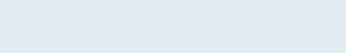# Sum Specific Text if Column Header Matches

After reading this article you will be able to sum specific numbers when text lookup value is given.

Adding the respective value of a particular name in a column based on the lookup value using the Excel’s Index and Match function. In case of similar names in a column and selecting one of the multiple rows or columns, we can apply this formula.

QUERY:

We have columns vendors and prices of other, fruit and vegetable. Our task is to know the position of fruit in the given range and then return the values of a matched column of the range. The purpose is to add all values (Price) in the selected range based on the given criteria of the vendor’s name. We will use the INDEX and MATCH along with SUMIF formula to add vendors price based on condition.

Formula to implement:

=SUMIF(\$B\$5:\$B\$15,G\$6,INDEX(\$C\$5:\$E\$15,,MATCH(H\$5,\$C\$4:\$E\$4,)))

Functions applicable:

We will be creating our formula with Index and Match along with SUMIF formula in Excel:

• MATCH: Searches for a specified item in a range of cells, and then returns the relative position of that item in the range.
• INDEX: Returns the value at a given position in a range or array. You can use the index to retrieve individual values or entire rows and columns.
• SUMIF: Excel categorizes SUMIF function under Math functions. This function adds all numbers in a range of cells that meet specific criteria. Criteria can be applied to dates, numbers and text using logical operators (>, <, <>, =) and wildcards (*, ?) for partial matching.

Formula Explanation (Step by Step)

Excel start calculating from the innermost parenthesis and then work outward. You can also assess debug formula using shortcut Key F9 function key.

Let’s break the formula into multiple small steps to understand its application.

First formula:

MATCH(H\$5,\$C\$4:\$E\$4,)Syntax: MATCH(lookup_value, lookup_array, [match_type])

Step 1:  MATCH(H\$5,\$C\$4:\$E\$4,)

Result: 2

It returns the position based on lookup value, here we have H5 i.e. “fruit” in the given range (C4 to E4) so it gives 2.

Second formula:

INDEX(\$C\$5:\$E\$15,, MATCH(H\$5,\$C\$4:\$E\$4,)))

Syntax: INDEX(array, row_num, [column_num])Step 2: INDEX(\$C\$5:\$E\$15,,2)Result:

Above result only return value of 2nd column as given position by step 1 (Index formula) i.e. ‘fruit’ after evaluating the cell range (C5:E15).

Integrate the first two formulas in the third formula.

Third formula:

SUMIF (\$B\$5:\$B\$15,G\$6, INDEX (\$C\$5:\$E\$15,,MATCH(H\$5,\$C\$4:\$E\$4,)Syntax:(range, criteria,[sum_range])

Step 3:
SUMIF (\$B\$5:\$B\$15,G\$6,\$D\$5:\$D\$15)

Result: \$6788

(1000+1941+925+1868+1054)

As we already know the position in step 1 and it returns all values in step 2 so, now it will add all the price of fruit in the given range (D5 to D15) that matches with the condition given in G6 i.e. “Stout”.

The formula is dynamic, and we can simply change the Price and Vendor name to update the result. You can also create a drop-down that will make this operation easy.